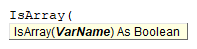# VBA IsArray Function

## IsArray Description

Used to check if a supplied variable is an array or not.

## Simple IsArray Examples

``````Dim a() As Integer
Debug.Print IsArray(a)``````

Result: True

``````Dim b As Integer
Debug.Print IsArray(b)``````

Result: False

## IsArray Syntax

In the VBA Editor, you can type  “IsArray(” to see the syntax for the IsArray Function:The IsArray function contains an argument:

VarName: A variable that will be evaluated.

## Examples of Excel VBA IsArray Function

``MsgBox IsArray("(1, 2, 3)")``

Result: False

``MsgBox IsArray(Array(1, 2, 3))``

Result: True

``````Sub IsArray_Example1()
Dim s As Variant
s = Split("A, B, C")
MsgBox IsArray(s)
End Sub``````

Result: True

Stop searching for VBA code online. Learn more about AutoMacro - A VBA Code Builder that allows beginners to code procedures from scratch with minimal coding knowledge and with many time-saving features for all users!